# Conjugate class of functions

A concept in the theory of functions which is a concrete instance of duality in functions spaces. Thus, if a classof functions is regarded as a Banach space or a topological vector space, then the conjugate class is defined as the class of functions isometrically isomorphic to the dual space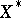. For example, whenand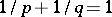, there is an isometric isomorphism between the spaces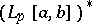and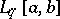, under which corresponding elements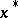andare related by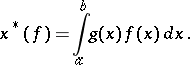If one considers some classof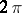-periodic summable functions on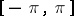, then the conjugate class is defined to be the class of functions conjugate to the functions in. For example, the class conjugate to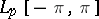(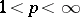) coincides with the class of functionsinfor which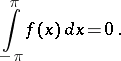The class conjugate to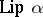,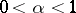, coincides with the class of functions infor which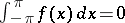.